# Worked Solutions 5: Numerical methods for ODEs: (a) explicit methods

In :
import numpy as np
import matplotlib.pyplot as plt
%matplotlib inline
plt.rcParams.update({'font.size': 14})  # Make the labels larger


### Question 1: Difference equations

In :
def question1a(h = 0.1, x0 = 0.2):
n = int(2/h)
ts = np.zeros(n+1)
xs = np.zeros(n+1)
xs = x0
for k in range(n):
ts[k+1] = ts[k] + h
xs[k+1] = xs[k] + 2*h*xs[k]**0.5
return ts, xs

# 1(a): various initial conditions
x0s = [0.0, 0.1, 0.2, 0.4, 0.6]
for x0 in x0s:
ts, xs = question1a(x0=x0)
plt.plot(ts, xs, '-+');
plt.xlabel('t'); plt.ylabel('x(t)'); plt.ylim(-0.1,8); plt.xlim(0,2);
# The x0=0 solution is an unstable equilibrium.

Out:
(0, 2)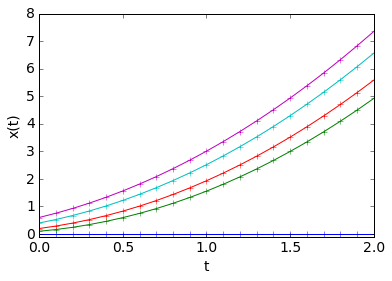Q1(b). Euler's method for an ODE $\frac{dx}{dt} = f(x,t)$ yields a difference equation $x_{k+1} = x_{k} + h \, f(x_k, t_k)$, where $h$ is the grid spacing.

Therefore, in this case $f(x,t) = 2 \sqrt{x}$. Thus, by separation of variables, $\int dt = \frac{1}{2} \int x^{-1/2} dx$ $\Rightarrow$ $t + c = x^{1/2}$ $\Rightarrow$ $x(t) = (t + \sqrt{x_0})^2$. ( Here $c = \sqrt{x_0}$ so that $x(t=0) = x_0$ ).

In :
# Question 1(c): The ratio test.
h0 = 0.025
# Try changing h0.  You should see that that the ratio gets closer to 2.
t0s, x0s = question1a(h=h0)
t1s, x1s = question1a(h=h0/2)
t2s, x2s = question1a(h=h0/4)
ratio = (x0s[1::1] - x1s[2::2])/(x1s[2::2] - x2s[4::4])
plt.plot(t0s[1:], ratio, 'r+-');
plt.xlabel("t"); plt.ylabel("ratio");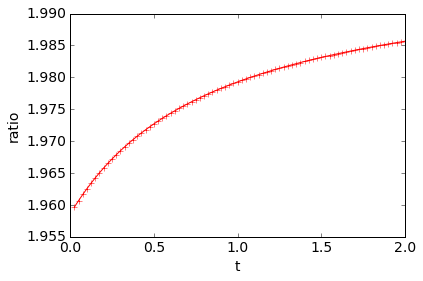Q1(d). The ratio takes the value of '2' because the ratio tends to $r \sim 2^s$, where $s$ is the order of accuracy of the method.

Euler's method is first-order accurate, therefore $s = 1$ and so $r \sim 2^1 = 2$.

### Question 2. The midpoint method.

In :
# Q2(a).
def dxdt(x,t):
return np.sin(t)**3
def midpoint(h = 0.1, x0 = -1.0):
tmax = 20.0
n = int(tmax/h)
ts = np.zeros(n+1)
xs = np.zeros(n+1)
xs = x0
for k in range(n):
xhalf = xs[k] + h/2*dxdt(xs[k], ts[k])
thalf = ts[k] + h/2
xs[k+1] = xs[k] + h*dxdt(xhalf, thalf)
ts[k+1] = ts[k] + h
return ts, xs
ts, xs = midpoint()
plt.plot(ts, xs, '-+');
plt.xlabel('t'); plt.ylabel('x(t)');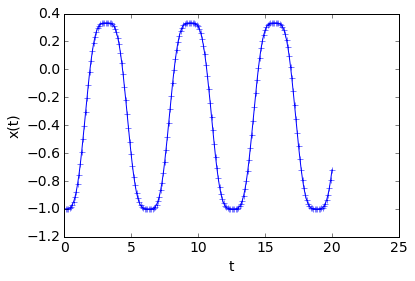In :
# Question 2(b): The ratio test.
h0 = 0.025
# Try changing h0.  You should see that that the ratio gets closer to 2.
t0s, x0s = midpoint(h=h0)
t1s, x1s = midpoint(h=h0/2)
t2s, x2s = midpoint(h=h0/4)
ratio = (x0s[1::1] - x1s[2::2])/(x1s[2::2] - x2s[4::4])
plt.plot(t0s[1:], ratio, 'r+-');
plt.xlabel("t"); plt.ylabel("ratio");
plt.ylim(0,5);
# When x(t) passes through zero, the ratio can 'spike', as the denominator in the ratio can pass through zero.
# The ratio is close to 4 = 2^2 because the midpoint method is second-order accurate.

Out:
(0, 5)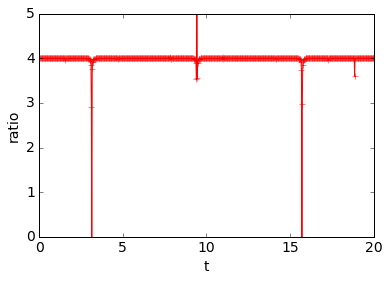In :
# Q2(c)
# Pass 'N' as an optional argument
def dxdtn(x,t,N=11):
return np.sin(t)**N
def midpoint(h = 0.1, x0 = -1.0, N=21):
tmax = 20.0
n = int(tmax/h)
ts = np.zeros(n+1)
xs = np.zeros(n+1)
xs = x0
for k in range(n):
xhalf = xs[k] + h/2*dxdtn(xs[k], ts[k], N)
thalf = ts[k] + h/2
xs[k+1] = xs[k] + h*dxdtn(xhalf, thalf, N)
ts[k+1] = ts[k] + h
return ts, xs
ts, xs = midpoint()
plt.plot(ts, xs, '-+');
plt.xlabel('t'); plt.ylabel('x(t)');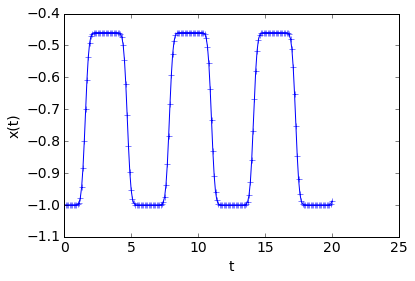In [ ]: